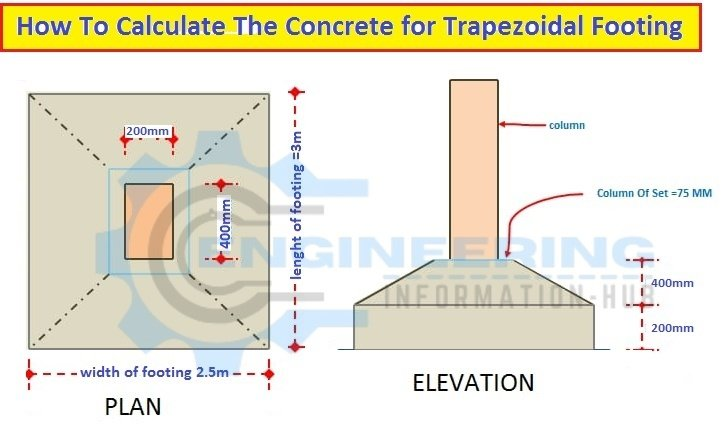# Calculate The Concrete for The Trapezoidal Footing

This article will calculate the volume of concrete for the trapezoidal footing | volume of concrete for the trapezoidal footing. What is the trapezoidal footing? How To Calculate The Concrete For Trapezoidal Footing

## What is the trapezoidal footing?

trapezoidal footing ould be the basic type of footing that carries unequal masses from two columns once the heaviest load outside the column distance is proscribed. it’s used once the area unit he worlds the realm of footing is mounted and also the soils at the location is loose soil.

### Example

Given dataWe will divide the footing into two portions: a rectangle and 2nd is a trapezoidal portion.

### 1st portion rectangle

Length =3m

Width=2.5m

Height = 0.200m

Now Calculate the volume of the rectangle portion

Volume of rectangle portion= length x width x height

Volume of rectangle portion= 3 x 2.5 x0.200=1.5m³

### 2nd portion

Length =0.400m

Width =0.200m

Height =0.400m

Now we calculate the volume of trapezoidal portion

Using formula    1/3 {A1 + A2 + (√A1 x A2)H}

Volume of trapezoidal portion = 1/3 {A1 + A2 + (√A1 x A2)H}

We need the area of rectangle portion and trapezoidal portion

We calculate the area of both portion

### Area of rectangle portion =A1

A1= length x width

A1 = 3  x  2.5

A1=7.5m²

### Area of 2nd portion = A2

A2 = length  x width

A2 =0.400 x 0.200

A2 = 0.08m²

Now put The value in formula

Volume of trapezoidal portion  = 1/3 {A1 + A2 + (√A1 x A2)H}

Volume of trapezoidal portion = 1/3 {7.5+0.08+((√7.5 x 0.08)0.4}

Volume of trapezoidal portion = 1/3(7.58+0.60)0.4

Volume of trapezoidal portion = 1/3(3.341)

Volume of trapezoidal portion 1.113m³

### Now the total volume of concrete for the trapezoidal footing

the volume of concrete for trapezoidal footing=  volume of rectangle +volume of trapezoidal

volume of concrete for trapezoidal footing=  1.500 +1.113 =2.613m3

### Raja Numan

Hi, My name is Engr. Raja Numan author of Engineering Information Hub and I am a Civil Engineer by Profession and I've specialized in the field of Quantity Surveying, Land Surveying as QC Engineer in national and multinational companies of Pakistan & Saudi Arabia.

### Related Articles

1.Fikre says: